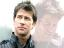# A Simple Analogical Clock (example)

8 replies to this topic

### #1Major

Major

Newbie

•• Members
•• 17 posts
• Location:Toulouse, France.

• Calculators:

Posted 06 April 2006 - 09:08 PM

Here is a small example of an analogical clock, feel free to create some other ones ! Orwell should soon add some new functions to get and set the current time, so it will be interesting to update this program and compare the changes !```require("draw")
showgraph()
fullscreen(0)

pi = math.pi

r, u, v, z = 50, 80, 100, 20000 // should adjust this last value for better precision...

for h = 0,24 do
for m = 0,60 do
for s = 0,60 do

x1, y1 = u+r*math.cos((s-15)*pi/30), v+r*math.sin((s-15)*pi/30)
x2, y2 = u+r*math.cos((m-15)*pi/30), v+r*math.sin((m-15)*pi/30)
x3, y3 = u+r*math.cos((h-15)*pi/30), v+r*math.sin((h-15)*pi/30)

draw.circle(u,v,r)
draw.circle(u,v,r+2)
draw.circle(u,v,3,3)
draw.line(u,v,x1,y1)
draw.line(u,v,2*(x2-u)/3+u,2*(y2-u)/3+u)
draw.line(u,v,(x3-u)/2+u,(y3-v)/2+v,1,2)

for i = 0,z do end

draw.line(u,v,x1,y1,0) end
draw.line(u,v,2*(x2-u)/3+u,2*(y2-u)/3+u,0) end
draw.line(u,v,(x3-u)/2+u,(y3-v)/2+v,0,2) end```

### #22072

2072

Casio over god

•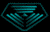•• 1564 posts
• Gender:Male
• Location:Somewherebourg
• Interests:Alternative states of consciousness, programming, making things work the best they possibly can.

• Calculators:
AFX2 ROM 1.02, CFX-9940GT+, FX-180P-Plus

Posted 07 April 2006 - 06:19 PM

oh a Stargate fan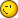### #3Tchernoben

Tchernoben

Casio Fan

•• Members
•• 42 posts
• Location:Nice
• Interests:Calculators, Pc, mangas, warhammer.

• Calculators:
Ti 89 Titanium

Posted 07 April 2006 - 07:24 PM

It's me or this program doesn't work ?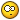You've forgoten :
```showgraph()
fullscreen(0)```

### #4Major

Major

Newbie

•• Members
•• 17 posts
• Location:Toulouse, France.

• Calculators:

Posted 08 April 2006 - 01:42 PM

Using the new function gettime(), here is a new version of my clock with several improvements.
Now the hour is automatically updated and the duration of one second is not defined by a constant anymore.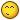```require("draw")

showgraph()
fullscreen(0)
draw.onbuffer()

pi = math.pi

repeat

h,m,s=gettime()

x1,y1=80+50*math.cos((s-15)*pi/30),100+50*math.sin((s-15)*pi/30)
x2,y2=80+50*math.cos((m-15)*pi/30),100+50*math.sin((m-15)*pi/30)
x3,y3=80+50*math.cos((h-15)*pi/30),100+50*math.sin((h-15)*pi/30)

draw.circle(80,100,52)
draw.circle(80,100,54)
draw.circle(80,100,3,3)
draw.line(80,100,x1,y1)
draw.line(80,100,2*(x2-80)/3+80,2*(y2-100)/3+100)
draw.line(80,100,(x3-80)/2+80,(y3-100)/2+100,1,2)
draw.pixel(80,100,0)
draw.rect(60,159,104,169)
draw.text(62,160,h..":"..m..":"..s)

draw.update()

draw.clear()

until testkey(K_EXE)```

### #5Major

Major

Newbie

•• Members
•• 17 posts
• Location:Toulouse, France.

• Calculators:

Posted 08 April 2006 - 01:53 PM

There will soon be a menu to define the correct time of the calculator; it is actually easy to do with settime() in the interactive mode for example.
I think I will upload the clock on the File Sharing after that.

### #6Major

Major

Newbie

•• Members
•• 17 posts
• Location:Toulouse, France.

• Calculators:

Posted 09 April 2006 - 12:04 PM

Okay, the first version of my analogical clock is now available on the file sharing area !I hope that you will like it and that it will be useful for you.

```require("draw")
require("string")

showgraph()
fullscreen(1)
draw.onbuffer()

pi = math.pi

repeat

h,m,s=gettime()
b,c,d=getdate()

if h>11 then h1=h-12 else h1=h end

h1=h1+m/60

str1=string.format("%02d:%02d:%02d",h,m,s)
str2=string.format("%02d",d)
str3=string.format("%02d/%02d/%02d",d,c,B)
x1,y1=80+50*math.cos((s-15)*pi/30),100+50*math.sin((s-15)*pi/30)
x2,y2=80+50*math.cos((m-15)*pi/30),100+50*math.sin((m-15)*pi/30)
x3,y3=80+50*math.cos((h1-3)*pi/6),100+50*math.sin((h1-3)*pi/6)

draw.circle(80,100,52)
draw.circle(80,100,54)
draw.circle(80,100,3,3)
draw.line(80,48,80,52)
draw.line(80,148,80,152)
draw.line(128,100,132,100)
draw.line(29,100,32,100)
draw.line(124,125.5,121,123.5)
draw.line(105.5,144,103.5,141)
draw.line(54.5,144,56.5,141)
draw.line(36,125.5,39,123.5)
draw.line(36,74.5,39,76.5)
draw.line(54.5,56,56.5,59)
draw.line(105.5,56,103.5,59)
draw.line(124,74.5,121,76.5)

draw.line(80,100,x1,y1)
draw.line(80,100,5*(x2-80)/6+80,5*(y2-100)/6+100)
draw.line(80,100,3*x3/5+32,3*y3/5+40,1,2)

draw.pixel(80,100,0)
draw.text(20,5,"Analogical Clock V1.0",2,10,1)
draw.text(63,156,str1,2,9)
draw.text(119,98,str2,1,6)
draw.text(63,165,str3,1,6)
draw.rect(21,42,139,173,2)
draw.update()
draw.clear()

if testkey(K_DOWN) then showconsole() print("	Analogical Clock for	  Classpad by Pierre Brian			 v1.0")
print(" ")
print("	 Press -> to exit")
waitkey(K_RIGHT) showgraph() clear() end
if testkey(K_UP) then showconsole() clear() print("Hour ?")
H=tonumber(input("Hour :"))
print(" ")
print("Minute ?")
M=tonumber(input("Minute :"))
print(" ")
settime(H,M)
showgraph() print("	 Press -> to exit")
clear()
end

until testkey(K_BACK)```

### #7Kilburn

Kilburn

Casio Technician

•• Members
•• 491 posts
• Gender:Male
• Location:France
• Interests:Blah

• Calculators:
FX-7500 G

Posted 12 April 2006 - 08:06 PM

You could make the minute and hour hands ("aiguilles") move progressively. Just add:

```h=h+m/60
m=m+s/60```

just after h,m,s=gettime(). This should look better.### #8Tchernoben

Tchernoben

Casio Fan

•• Members
•• 42 posts
• Location:Nice
• Interests:Calculators, Pc, mangas, warhammer.

• Calculators:
Ti 89 Titanium

Posted 12 April 2006 - 09:38 PM

Hey not bad at allUnfortunately my classpad crash too many times (everytime fatals errors exeptions) so I don't know if time will be always the same### #9Major

Major

Newbie

•• Members
•• 17 posts
• Location:Toulouse, France.

• Calculators:
I'm working on a new version with Kilburn's idea (I must thank you for that); you will also be able to set the time directly by moving the hands. I think it will be nice and more useful !